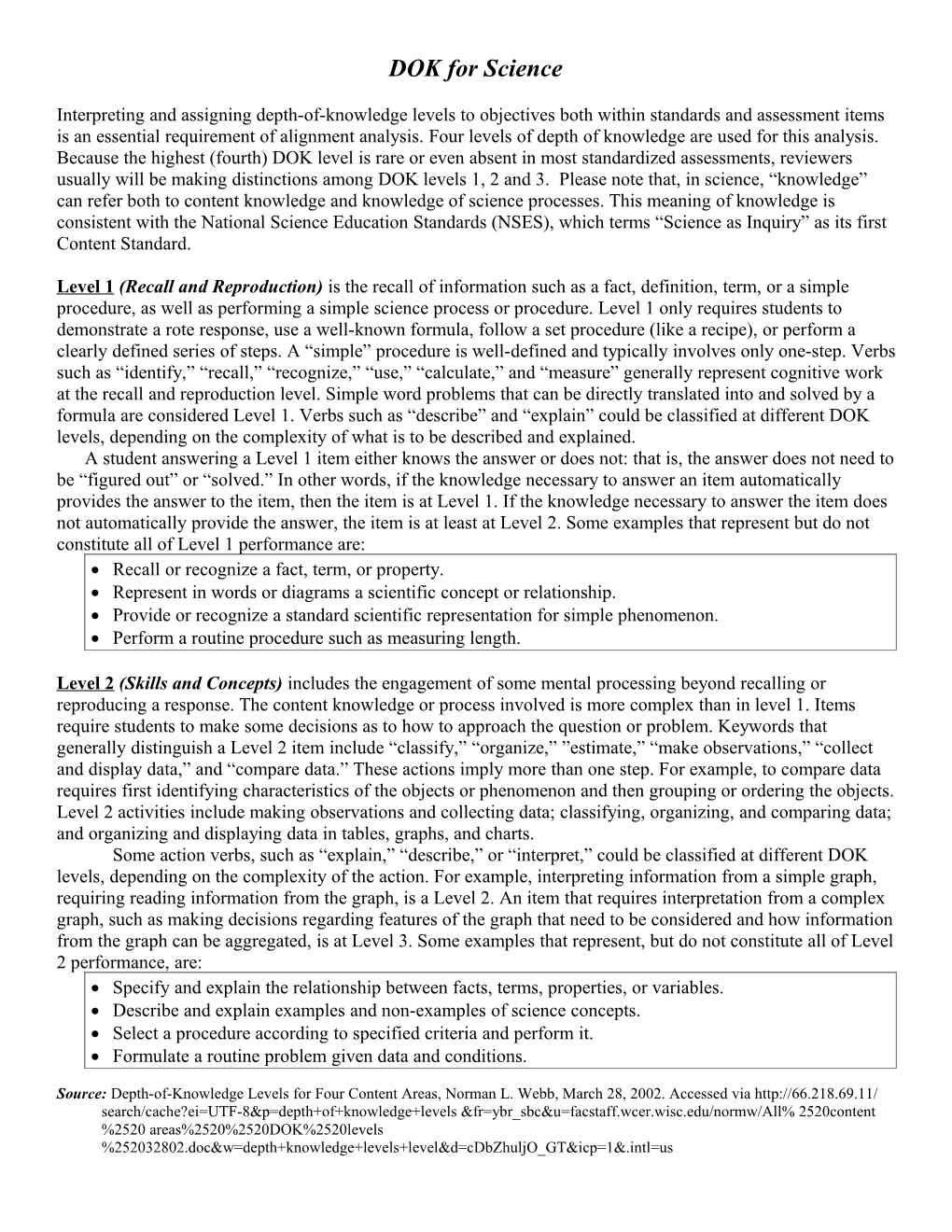# DOK for Reading (Based on Wixson, 1999)DOK for Science

Interpreting and assigning depth-of-knowledge levels to objectives both within standards and assessment items is an essential requirement of alignment analysis. Four levels of depth of knowledge are used for this analysis. Because the highest (fourth) DOK level is rare or even absent in most standardized assessments, reviewers usually will be making distinctions among DOK levels 1, 2 and 3. Please note that, in science, “knowledge” can refer both to content knowledge and knowledge of science processes. This meaning of knowledge is consistent with the National Science Education Standards (NSES), which terms “Science as Inquiry” as its first Content Standard.

Level 1(Recall and Reproduction) is the recall of information such as a fact, definition, term, or a simple procedure, as well as performing a simple science process or procedure. Level 1 only requires students to demonstrate a rote response, use a well-known formula, follow a set procedure (like a recipe), or perform a clearly defined series of steps. A “simple” procedure is well-defined and typically involves only one-step. Verbs such as “identify,” “recall,” “recognize,” “use,” “calculate,” and “measure” generally represent cognitive work at the recall and reproduction level. Simple word problems that can be directly translated into and solved by a formula are considered Level 1. Verbs such as “describe” and “explain” could be classified at different DOK levels, depending on the complexity of what is to be described and explained.

A student answering a Level 1 item either knows the answer or does not: that is, the answer does not need to be “figured out” or “solved.” In other words, if the knowledge necessary to answer an item automatically provides the answer to the item, then the item is at Level 1. If the knowledge necessary to answer the item does not automatically provide the answer, the item is at least at Level 2. Some examples that represent but do not constitute all of Level 1 performance are:

• Recall or recognize a fact, term, or property.
• Represent in words or diagrams a scientific concept or relationship.
• Provide or recognize a standard scientific representation for simple phenomenon.
• Perform a routine procedure such as measuring length.

Level 2(Skills and Concepts) includes the engagement of some mental processing beyond recalling or reproducing a response. The content knowledge or process involved is more complex than in level 1. Items require students to make some decisions as to how to approach the question or problem. Keywords that generally distinguish a Level 2 item include “classify,” “organize,” ”estimate,” “make observations,” “collect and display data,” and “compare data.” These actions imply more than one step. For example, to compare data requires first identifying characteristics of the objects or phenomenon and then grouping or ordering the objects. Level 2 activities include making observations and collecting data; classifying, organizing, and comparing data; and organizing and displaying data in tables, graphs, and charts.

Some action verbs, such as “explain,” “describe,” or “interpret,” could be classified at different DOK levels, depending on the complexity of the action. For example, interpreting information from a simple graph, requiring reading information from the graph, is a Level 2. An item that requires interpretation from a complex graph, such as making decisions regarding features of the graph that need to be considered and how information from the graph can be aggregated, is at Level 3. Some examples that represent, but do not constitute all of Level 2 performance, are:

• Specify and explain the relationship between facts, terms, properties, or variables.
• Describe and explain examples and non-examples of science concepts.
• Select a procedure according to specified criteria and perform it.
• Formulate a routine problem given data and conditions.
• Organize, represent and interpret data.

Level 3(Strategic Thinking) requires reasoning, planning, using evidence, and a higher level of thinking than the previous two levels. The cognitive demands at Level 3 are complex and abstract. The complexity does not result only from the fact that there could be multiple answers, a possibility for both Levels 1 and 2, but because the multi-step task requires more demanding reasoning. In most instances, requiring students to explain their thinking is at Level 3; requiring a very simple explanation or a word or two should be at Level 2. An activity that has more than one possible answer and requires students to justify the response they give would most likely be a Level 3. Experimental designs in Level 3 typically involve more than one dependent variable. Other Level 3 activities include drawing conclusions from observations; citing evidence and developing a logical argument for concepts; explaining phenomena in terms of concepts; and using concepts to solve non-routine problems. Some examples that represent, but do not constitute all of Level 3 performance, are: